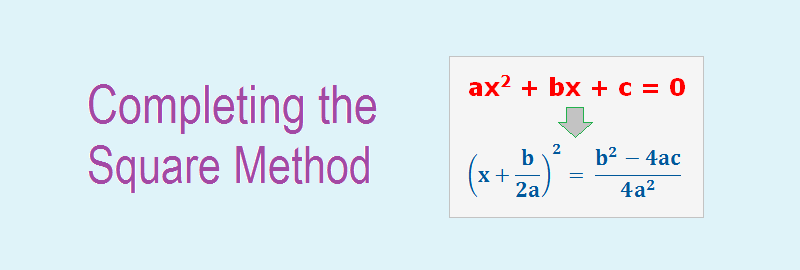# Completing the Square Method## Completing the Square Method

In completing the square method for solving a quadratic equation of the form ax2 + bx + c = 0, we transpose the constant term c to the right hand side of the equation and then the left hand side is expressed as a perfect square expression.

************************************

10 Math Problems officially announces the release of Quick Math Solver, an android APP on the Google Play Store for students around the world.

**************************

We use the following steps for solving quadratic equation of the form ax2 + bx + c = 0 by completing the square method:

#### Steps:

1.  Rewrite the equation so that the terms containing x2 and x (i.e. variable) are on the left side and the constant term is on the right side.
2.  If the coefficient of x2 is other than 1, then reduce the coefficient of x2 as 1 by dividing on both sides by the coefficient of x2.
3.  Add the square of half of the coefficient of x on both sides of the equation to make the left hand side of the equation a complete square.
4.  Express the left hand side of the equation as a perfect square of a binomial.
5.  Use the square root property on both sides and calculate the roots.

This process of Completing the Square Method will be clear by the following worked out examples.

### Workout Examples

Example 1: Solve the following quadratic equations by completing the square method.
a.    x2 – 2x – 8 =0
b.    2x2 + 5x – 12 = 0
c.     3x2 – 5x + 2 = 0

Solution:  a) Here,
x2 – 2x – 8 = 0
or,     x2 – 2x = 8
or,     x2 – 2x + 1 = 8 + 1  [adding both sides by 1]
or,     (x – 1)2 = 9
or,     x – 1 = ±√9
or,     x – 1 = ±3
or,     x = 1 ± 3
Taking +ve sign,
x = 1 + 3 = 4
Taking –ve sign,
x = 1 – 3 = 2
x = 4, 2

Solution:  b) Here,
2x2 + 5x – 12 = 0
or,     2x2 + 5x = 12
or,     (2x2 + 5x)/2 = 12/2  [dividing both sides by 2]
or,     x2 +5x/2 = 6
or,     x2 + 2.x.5/4 + (5/4)2 = 6 + (5/4)2  [adding both sides by (5/4)2]
or,     (x + 5/4)2 = 6 + 25/16
or,     (x + 5/4)2 = 121/16
or,     x + 5/4 = ±√(121/16)
or,     x + 5/4 = ± 11/4
or,     x = 5/4 ± 11/4
Taking +ve sign,
x = 5/4 + 11/4 = (5 + 11)/4 = 6/4 = 3/2
Taking –ve sign,
x = 5/4 11/4 = (–5 – 11)/4 = –16/4 = –4
x = 3/2, –4

Solution:  c) Here,
3x2 – 5x + 2 = 0
or,     3x2 5x = 2
or,     (3x2 5x)/3 = 2/3  [dividing both sides by 3]
or,     x2 5x/3 = 2/3
or,     x2 2.x.5/6 + (5/6)2 = 2/3 + (5/6)2  [adding both sides by (5/6)2]
or,     (x 5/6)2 = 2/3 + 25/36
or,     (x 5/6)2 = (24 + 25)/36
or,     x 5/6 = ±√(1/36)
or,     x 5/6 = ± 1/6
or,     x = 5/6 ± 1/6
Taking +ve sign,
x = 5/6 + 1/6 = (5 + 1)/6 = 6/6 = 1
Taking –ve sign,
x = 5/6 1/6 = (5 –1)/6 = 4/6 = 2/3
x = 1, 2/3

You can comment your questions or problems on solving quadratic equations by ‘completing the square method’ here.